# 5 Verification of the structural elements according to Eurocode

Tento článek je dostupný také v dalších jazycích:
$$Assessment of the structure using the CSFM is performed by two different analyses: one for serviceability, and one for ultimate limit state load combinations. The serviceability analysis assumes that the ultimate behavior of the element is satisfactory, and the yield conditions of the material will not be reached at serviceability load levels. This approach enables the use of simplified constitutive models (with a linear branch of concrete stress-strain diagram) for serviceability analysis to enhance numerical stability and calculation speed.5.1 Materials5.1.1 Concrete - ULSThe concrete model implemented in the CSFM is based on the uniaxial compression constitutive laws prescribed by EN 1992-1-1 for the design of cross-sections, which only depend on compressive strength. The parabola-rectangle diagram specified in EN 1992-1-1 (Fig. 26a) is used by default in the CSFM, but designers can also choose a more simplified elastic ideal plastic relationship (Fig. 26b). The tensile strength is neglected, as it is in classic reinforced concrete design.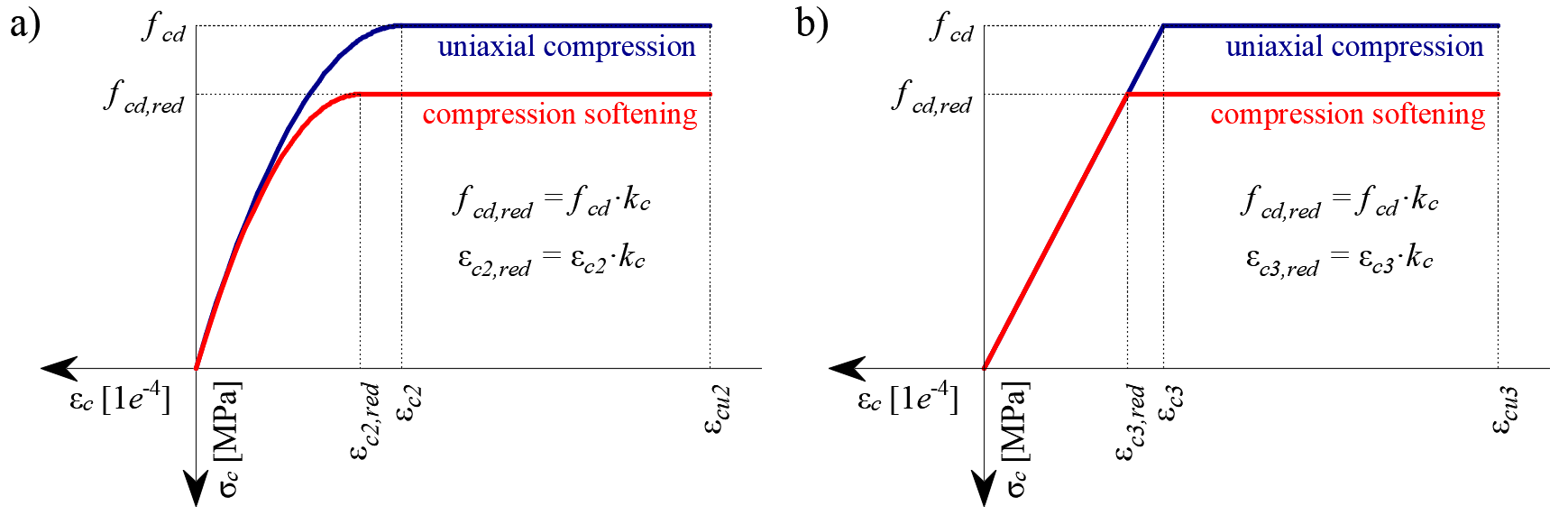$\textsf{\textit{\footnotesize{Fig. 26\qquad The stress-strain diagrams of concrete for ULS: a) parabola-rectangle diagram; b) bilinear diagram.}}}$Using default settings, the current implementation of the CSFM in IDEA StatiCa Detail does not consider an explicit failure criterion in terms of strains for concrete in compression (i.e., after the peak stress is reached it considers a plastic branch with εcu2 (εcu3) in value 5% while EN 1992-1-1 assumes ultimate strain less than 0,35%). This simplification does not allow the deformation capacity of structures failing in compression to be verified. However, their ultimate capacity is properly predicted when, in addition to the factor of cracked concrete (kc2 defined in (Fig. 27)), the increase in the brittleness of concrete as its strength rises is considered by means of the $$\eta_{fc}$$ reduction factor defined in fib Model Code 2010 as follows:$f_{cd}=\frac{f_{ck,red}}{γ_c} = \frac{k_c \cdot f_{ck}}{γ_c} = \frac{\eta _{fc} \cdot k_{c2} \cdot f_{ck}}{γ_c}$${\eta _{fc}} = {\left( {\frac{{30}}{{{f_{ck}}}}} \right)^{\frac{1}{3}}} \le 1$where:kc is the global reduction factor of the compressive strengthkc2 is the reduction factor due to the presence of transverse crackingfck is the concrete cylinder characteristic strength (in MPa for the definition of $$\eta_{fc}$$).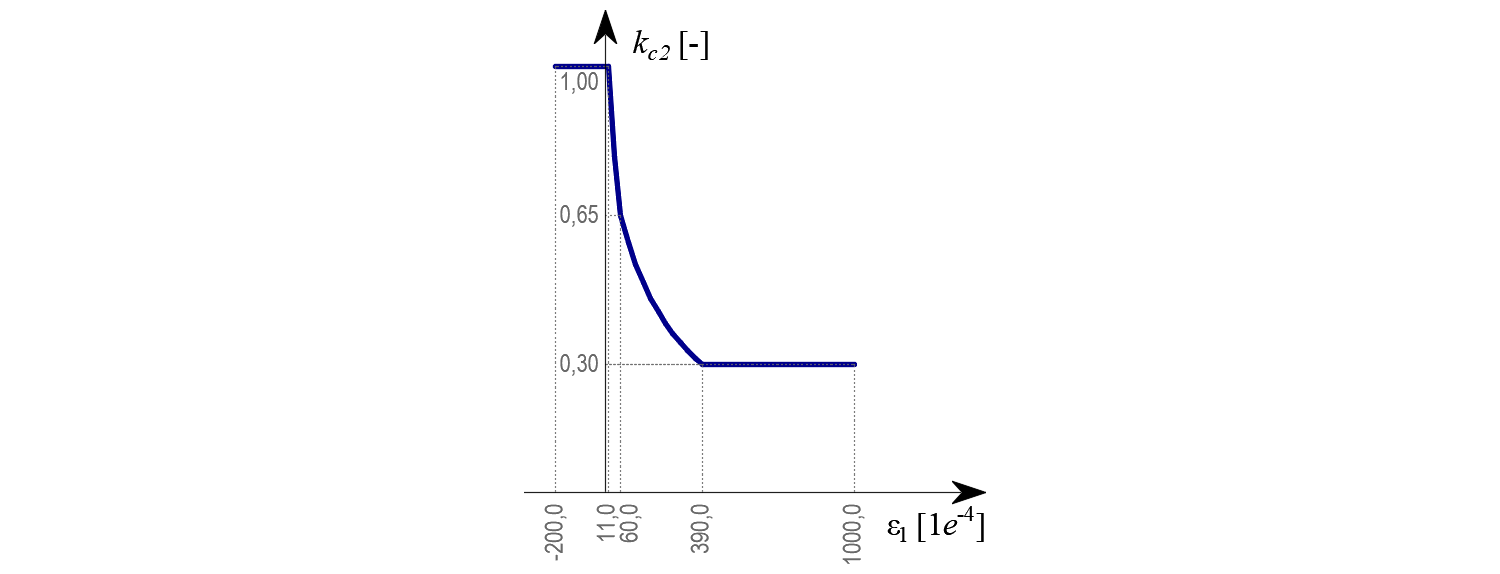$\textsf{\textit{\footnotesize{Fig. 27\qquad The compression softening law.}}}$5.1.2 Concrete - SLSThe serviceability analysis contains certain simplifications of the constitutive models which are used for ultimate limit state analysis. A perfect bond is assumed, i.e., the anchorage length is not verified at serviceability. Furthermore, the plastic branch of the stress-strain curve of concrete in compression is disregarded, while the elastic branch is linear and infinite. Compression softening law is not considered. These simplifications enhance the numerical stability and calculation speed and do not reduce the generality of the solution as long as the resultant material stress limits at serviceability are clearly below their yielding points (as required by Eurocode). Therefore, the simplified models used for serviceability are only valid if all verification requirements are fulfilled.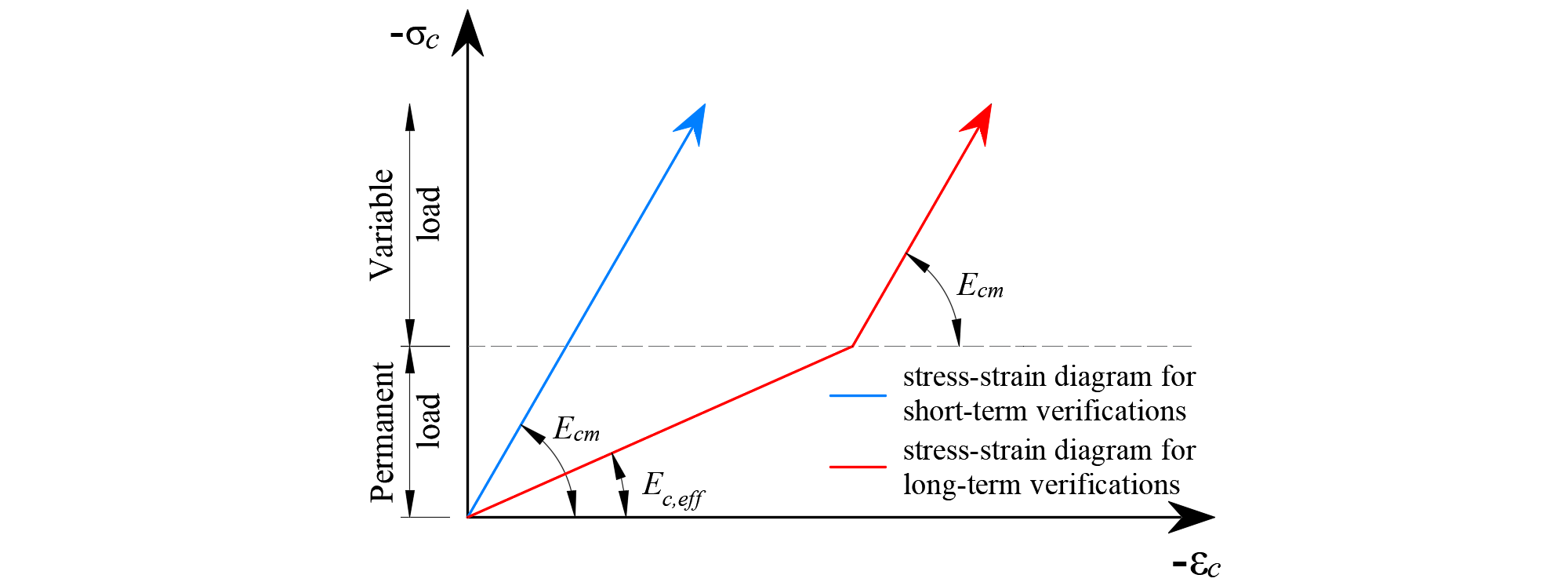$\textsf{\textit{\footnotesize{Fig. 28\qquad Concrete stress-strain diagrams implemented for serviceability analysis: short- and long-term verifications.}}}$Long term effectsIn serviceability analysis, the long-term effects of concrete are considered using an effective infinite creep coefficient ($$\varphi$$, taken as a value of 2.5 by default) which modifies the secant modulus of elasticity of concrete (Ecm) as follows:$E_{c,eff} = \frac{E_{cm}}{1+\varphi}$When considering long-term effects, a load step with all permanent loads is first calculated considering the creep coefficient (i.e., using the effective modulus of elasticity of concrete, Ec,eff) and, then, the additional loads are calculated without the creep coefficient (i.e., using Ecm). In addition, to conduct short-term verifications, another calculation is performed in which all loads are calculated without the creep coefficient. Both calculations for long and short-term verifications are depicted in Fig. 28.5.1.3 ReinforcementBy default, the idealized bilinear stress-strain diagram for the bare reinforcing bars typically defined by design codes (Fig. 29) is considered. The definition of this diagram only requires basic properties of the reinforcement to be known during the design phase (strength and ductility class). Whenever known, the actual stress-strain relationship of the reinforcement (hot-rolled, cold-worked, quenched and self-tempered, …) can be considered. The reinforcement stress-strain diagram can be defined by the user, but in this case, it is impossible to assume tension stiffening effect (it is impossible to calculate crack width). Using the stress-strain diagram with horizontal top branch does not allow to the verification of structural durability. Therefore, manual verification of standard ductility requirements is necessary.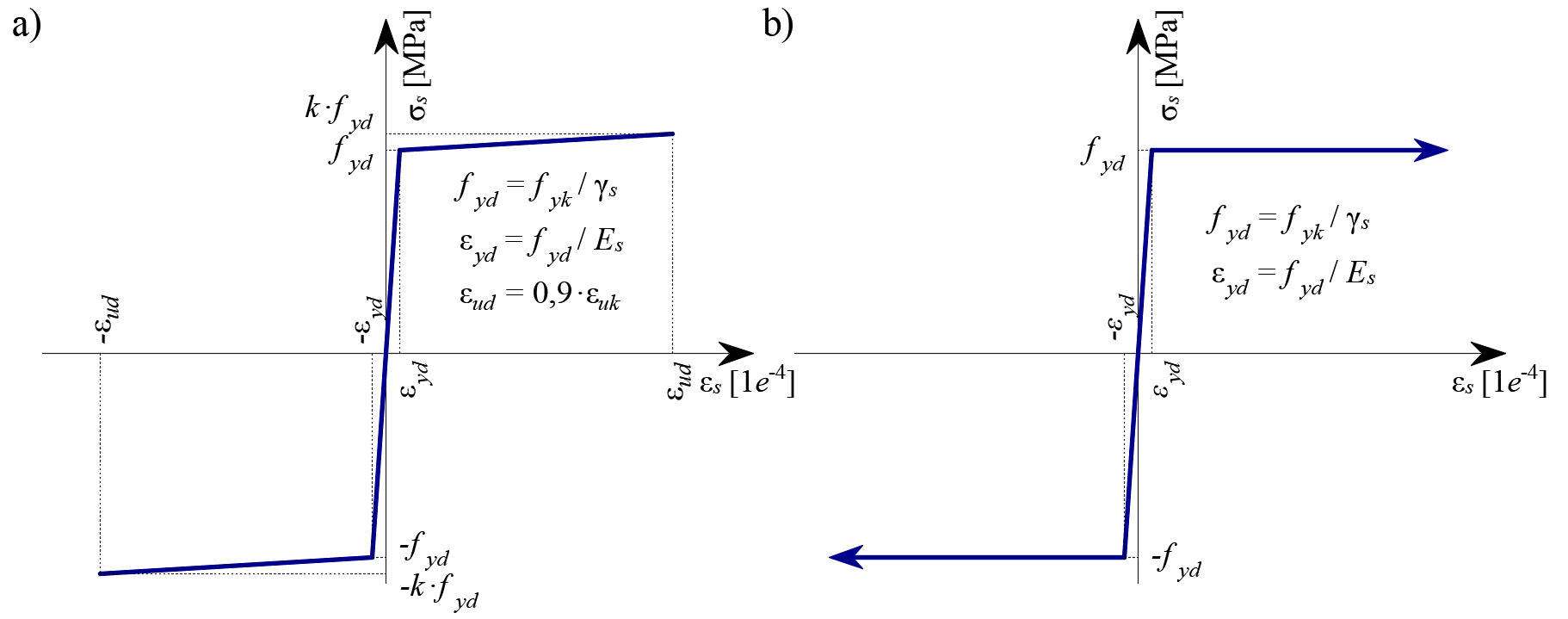$$\textsf{\textit{\footnotesize{Fig. 29 \qquad Stress-strain diagram of reinforcement: a) bilinear diagram with an inclined top branch; b) bilinear diagram}}}$$ $$\textsf{\textit{\footnotesize{with a horizontal top branch.}}}$$Tension stiffening (Fig. 30) is accounted for automatically by modifying the input stress-strain relationship of the bare reinforcing bar in order to capture the average stiffness of the bars embedded in the concrete (εm), following the approaches presented in Section 1.2.4.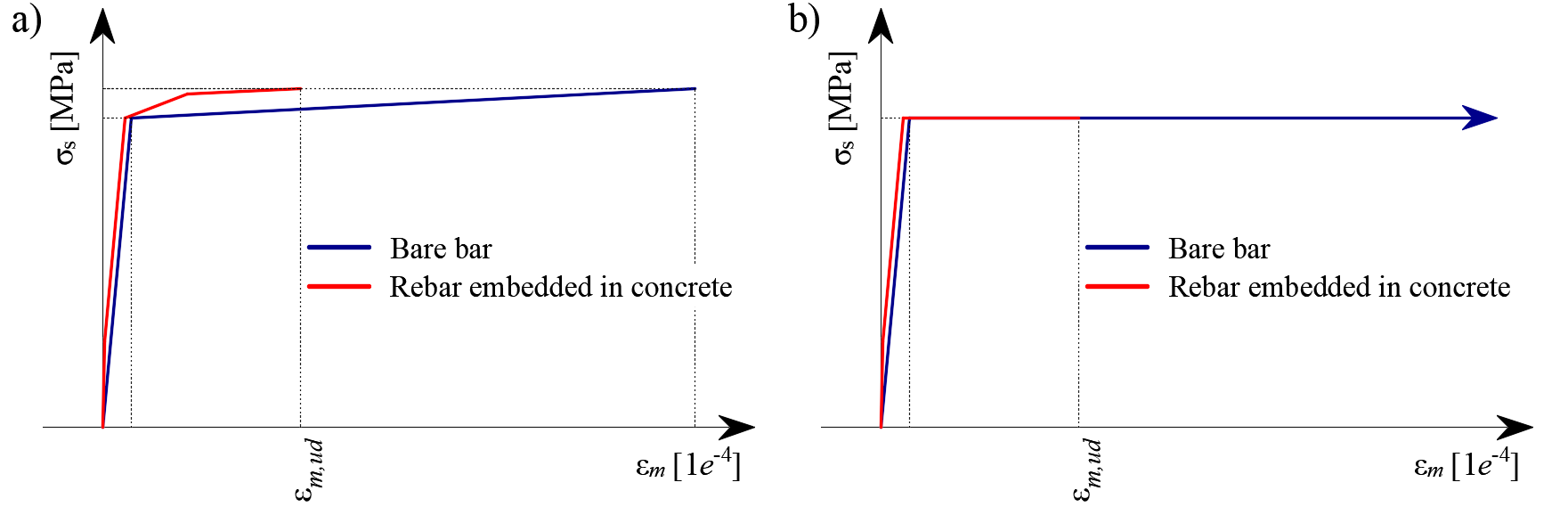$\textsf{\textit{\footnotesize{Fig. 30\qquad Scheme of tension stiffening.}}}$5.2 Safety format factorThe Compatible Stress Field Method is compliant with modern design codes. As the calculation models only use standard material properties, the partial safety factor format prescribed in the design codes can be applied without any adaptation. In this way, the input loads are factored and the characteristic material properties are reduced using the respective safety coefficients prescribed in design codes, exactly as in conventional concrete analysis. Values of material safety factors prescribed in EN 1992-1-1 chap. 2.4.2.4 are set by default, but user can change safety factors in Setup (Fig. 31).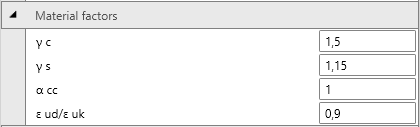$\textsf{\textit{\footnotesize{Fig. 31\qquad The setting of material safety factors in Idea StatiCa Detail.}}}$Load safety factors has to be defined by user in Combination rule for each non-linear combination of load cases (Fig. 32). For all templates implemented in Idea StatiCa Detail, partial safety factors are already predefined.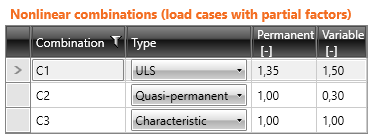$\textsf{\textit{\footnotesize{Fig. 32\qquad The setting of load partial factors in Idea StatiCa Detail.}}}$By using appropriate user-defined combinations of partial safety factors, users can also compute with the CSFM using the global resistance factor method (Navrátil, et al. 2017), but this approach is hardly ever used in design practice. Some guidelines recommend using the global resistance factor method for non-linear analysis. However, in simplified non-linear analyses (such as the CSFM), which only require those material properties that are used in conventional hand calculations, it is still more desirable to use the partial safety format.5.3 Ultimate limit state analysisThe different verifications required by EN 1992-1-1 are assessed based on the direct results provided by the model. ULS verifications are carried out for concrete strength, reinforcement strength, and anchorage (bond shear stresses).The concrete strength in compression is evaluated as the ratio between the maximum principal compressive stress σc3 obtained from FE analysis and the limit value σc3,lim. Then:$\frac{σ_{c3}}{σ_{c3,lim}}$$σ_{c3,lim} = f_{cd} = α_{cc} \cdot \frac{f_{ck,red}}{γ_c} = α_{cc} \cdot \frac{k_c \cdot f_{ck}}{γ_c} = α_{cc} \cdot \frac{\eta _{fc} \cdot k_{c2} \cdot f_{ck}}{γ_c}$${\eta _{fc}} = {\left( {\frac{{30}}{{{f_{ck}}}}} \right)^{\frac{1}{3}}} \le 1$where:fck characteristic cylinder strength of concrete,kc2 compression softening factor (see 5.1.1),γc is the partial safety factor for concrete, γc = 1,5,αcc is the coefficient taking account of long term effects on the compressive strength and of unfavourable effects resulting from the way the load is applied. The default value is 1,0. The strength of the reinforcement is evaluated in both tension and compression as the ratio between the stress in the reinforcement at the cracks σsr and the specified limit value σsr,lim:$\frac{σ_{sr}}{σ_{sr,lim}}$$$σ_{c3,lim} = \frac{k \cdot f_{yk}}{γ_s}\qquad\qquad\textsf{\small{for bilinear diagram with inclined top branch}}$$$$σ_{c3,lim} = \frac{f_{yk}}{γ_s}\qquad\qquad\,\,\,\,\textsf{\small{for bilinear diagram with horizontal top branch}}$$where:fyk yield strength of the reinforcement,k the ratio of tensile strength ftk to the yield stress, $$k = \frac{f_{tk}}{f_{yk}}$$γs is the partial safety factor for reinforcement γs = 1,15.The bond shear stress is evaluated independently as the ratio between the bond stress τb calculated by FE analysis and the ultimate bond strength fbd, according to EN 1992-1-1 chap. 8.4.2:$\frac{τ_{b}}{f_{bd}}$$f_{bd} = 2.25 \cdot η_1\cdot η_2\cdot f_{ctd}$where: fctd is the design value of concrete tensile strength. Due to the increasing brittleness of higher strength concrete, fctk,0,05 is limited to the value for C60/75, η1 is a coefficient related to the quality of the bond condition and the position of the bar during concreting (Fig. 33): η1 = 1,0 when ‘good’ conditions are obtained and η1 = 0,7 for all other cases and for bars in structural elements built with slip-forms, unless it can be shown that ‘good’ bond conditions existη2 is related to the bar diameter: η2 = 1,0 for Ø ≤ 32 mm η2 = (132 - Ø)/100 for Ø > 32 mm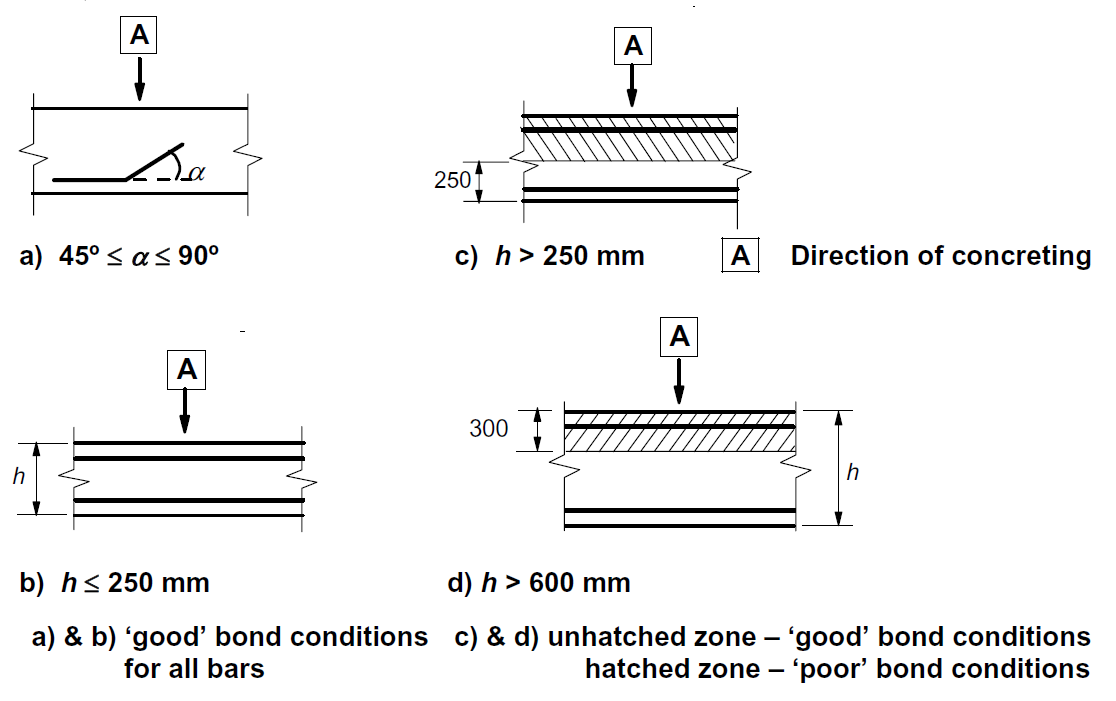$\textsf{\textit{\footnotesize{Fig. 33\qquad Description of bond conditions.}}}$These verifications are carried out with respect to the appropriate limit values for the respective parts of the structure (i.e., in spite of having a single grade both for concrete and reinforcement material, the final stress-strain diagrams will differ in each part of the structure due to tension stiffening and compression softening effects).5.4 Partially loaded areasWhen designing concrete structures, we meet two large groups of partially loaded areas (PLA) - the first of these comprises bearings, while the other consists of anchoring areas. According to currently valid standards for the design of reinforced concrete structures EN 1992-1-1 chap. 6.7 (Fig. 34), local crushing of concrete and transverse tension forces should be considered for partially loaded areas. For a uniformly distributed load on an area, Ac0, the compressive capacity of concrete may be increased by up to three times depending on the design distribution area, Ac1 (according to the new Eurocode concept, it is possible to increase load-bearing capacity up to seven times).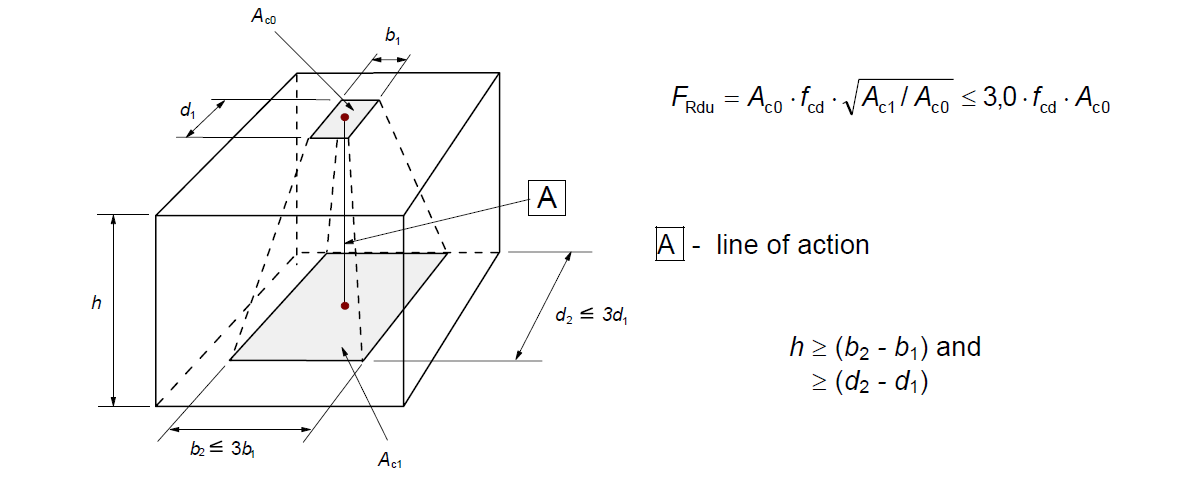$\textsf{\textit{\footnotesize{Fig. 34\qquad Partially loaded areas according to EN 1992-1-1.}}}$The partially loaded area must be sufficiently reinforced with transverse reinforcement designed to transmit the bursting forces that occur in the area. For the design of transverse reinforcement in partially loaded areas, the Strut-and-Tie method is used according to the Eurocode. Without the required transverse reinforcement, it is not possible to consider increasing the compressive capacity of the concrete.Partially loaded areas in the CSFM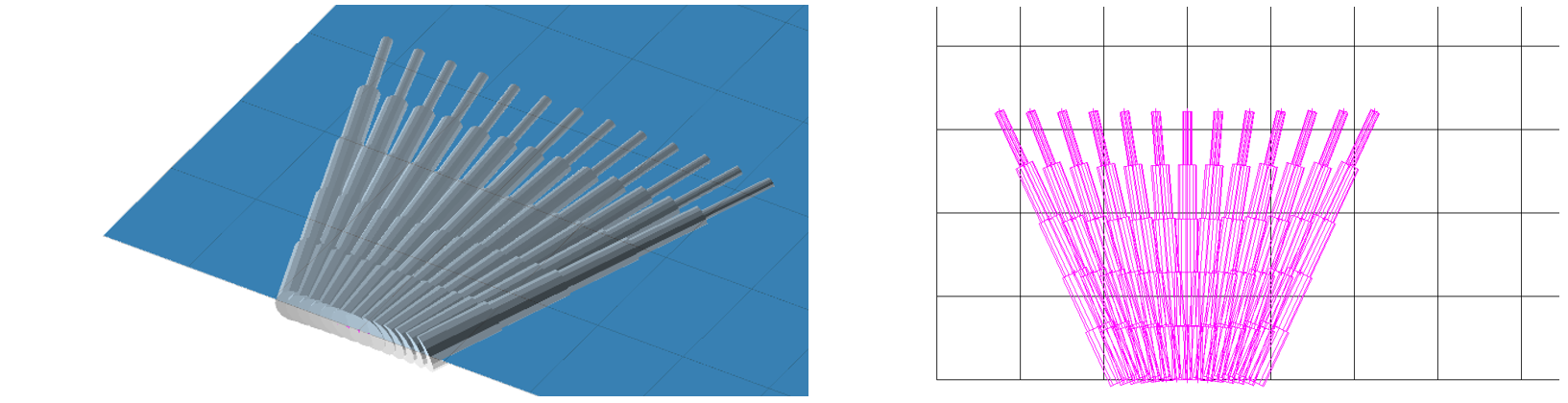$\textsf{\textit{\footnotesize{Fig. 35\qquad Fictitious struts with concrete finite element mesh.}}}$Using the CSFM, it is possible to design and assess reinforced concrete structures while including the influence of the increasing compressive resistance of concrete in partially loaded areas. Because the CSFM is a wall (2D) model and the partially loaded areas are a spatial (3D) task, it was necessary to find a solution that combines these two different types of tasks (Fig. 35). If the “partially loaded areas” function is activated, the allowable cone geometry is created according to the Eurocode (Fig. 34). All geometric collisions are solved fully in 3D for the specified concrete member geometry and the dimensions of each PLA. Subsequently, a computational model of the partially loaded area is created.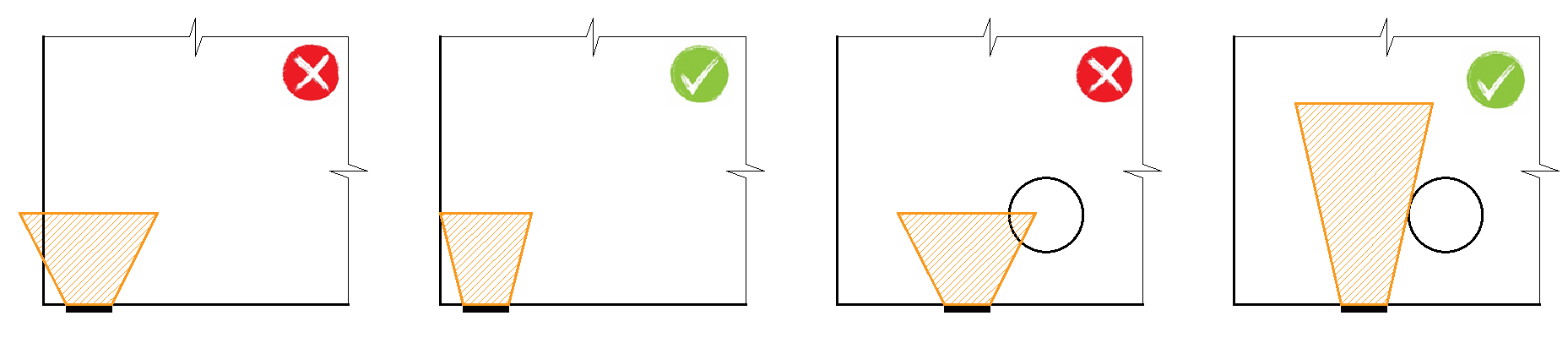$\textsf{\textit{\footnotesize{Fig. 36\qquad Allowable cone geometries.}}}$The modification of the material model proved to be an unsuitable approach, which was mainly because the mapping of properties to the finite element mesh is problematic. It was determined that an approach independent of the finite element mesh is a more appropriate solution. Absolutely coherent fictitious struts are created for the known compression cone geometry (Fig. 35 and Fig. 37). These struts have identical material properties to the concrete used in the model, including the stress-strain diagram. The shape of the cone determines the direction of the struts, which gradually distributes the load over the PLA to the design distribution area. The area density of the fictitious struts is variable at each part of the cone, and it adds a fictitious concrete area in the load direction. At the level of the loaded area (Ac0), a fictitious area of concrete is added according to the ratio $$\sqrt{A_{c0} \cdot A_{c1}} - A_{real}$$ (where Areal is an area of the support assumed in the 2D computational model), and this area decreases linearly to zero towards the design distribution area (Ac1). This solution ensures that the compressive stress in the concrete is constant over the entire cone volume.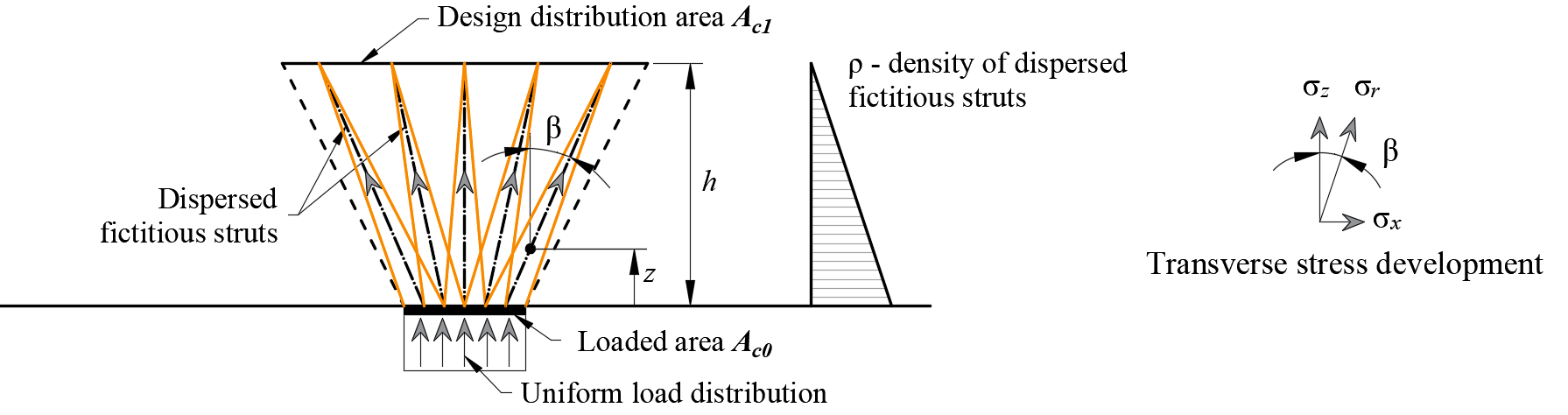$\rho \left( {\beta ,z} \right) = \left( {\sqrt {\frac{A_{c1}}{A_{c0}}} - \frac{A_{real}}{A_{c0}}} \right)\,\cdot\,\left( {1 - \frac{z}{h}} \right)\,\cdot\,\frac{1}{{\cos \beta }}$$\textsf{\textit{\footnotesize{Fig. 37\qquad Fictitious struts in the computational model}}}$The resistance of the partially loaded area is increased according to the ratio of the design distributed area and the loaded area laid in EN 1992-1-1 (6.7). It should be remembered that this is a design model that cannot precisely describe the stress state over a partially loaded area whose actual flow is much more complicated. However, this solution allows the correct distribution of load to the whole model while respecting the increased load capacity of the partially loaded area. In addition, it correctly introduces transverse stresses in this area.5.5 Serviceability limit state analysisSLS assessments are carried out for stress limitation, crack width and deflection limits. Stresses are checked in concrete and reinforcement elements according to EN 1992-1-1 in a similar manner to that specified for the ULS.5.5.1 Stress limitationThe compressive stress in the concrete shall be limited in order to avoid longitudinal cracks. According to EN 1992-1-1 chap. 7.2 (2), longitudinal cracks may occur if the stress level under the characteristic combination of loads exceeds a value k1fck. The concrete stress in compression is evaluated as the ratio between the maximum principal compressive stress σc3 obtained from FE analysis for serviceability limit states and the limit value σc3,lim. Then:$\frac{σ_{c3}}{σ_{c3,lim}}$$σ_{c3,lim} = k_1\cdot f_{ck}$where:fck characteristic cylinder strength of concrete,k1 =0.6.Unacceptable cracking or deformation may be assumed to be avoided if, under the characteristic combination of loads, the tensile stress in the reinforcement does not exceed k3fyk (EN 1992-1-1 chap. 7.2 (5)). The strength of the reinforcement is evaluated as the ratio between the stress in the reinforcement at the cracks σsr and the specified limit value σsr,lim:$\frac{σ_{sr}}{σ_{sr,lim}}$$σ_{sr,lim} = k_3\cdot f_{yk}$where:fyk yield strength of the reinforcement,k3 =0.8.5.5.2 DeflectionDeflections can only be assessed for walls or isostatic (statically determinate) or hyperstatic (statically indeterminate) beams. In these cases, the absolute value of deflections is considered (compared to the initial state before loading) and the maximum admissible value of deflections must be set by the user. Deflections at trimmed ends cannot be checked since these are essentially unstable structures where the equilibrium is satisfied by adding end forces and hence deflections are unrealistic. Short-term uz,st or long-term uz,lt deflection can be calculated and checked against user-defined limit values:$\frac{u_ z}{u_{z,lim}}$where:uz short- or long-term deflection calculated by FE analysis,uz,lim limit value of the deflection defined by the user.5.5.3 Crack widthCrack widths and crack orientations are calculated only for permanent loads, either short-term or long-term. The verifications with respect to limit values specified by the user according to the Eurocode are presented as follows:$\frac{w_ z}{w_{z,lim}}$where:w short- or long-term crack width calculated by FE analysis,wlim limit value of the crack width defined by the user.As presented in section 4.2.1, there are two ways of computing crack widths (stabilized and non-stabilized cracking). In the general case (stabilized cracking), the crack width is calculated by integrating the strains on 1D elements of reinforcing bars. The crack direction is then calculated from the three closest (from the center of the given 1D finite element of reinforcement) integration points of 2D concrete elements. While this approach to calculating the crack directions does not correspond to the real position of the cracks, it still provides representative values that lead to crack width results that can be compared to code-required crack width values at the position of the reinforcing bar.$$

## Vyzkoušejte program IDEA StatiCa

Stáhněte si zdarma zkušební verzi na 14 dní.